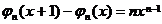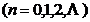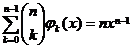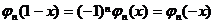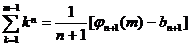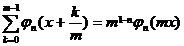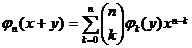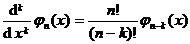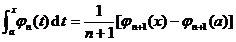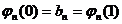§ 7   Bernoulli numbers and Bernoulli polynomials

1.        Bernoulli number

function expansion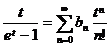In , the coefficients of , are called Bernoulli numbers, and are sometimes expanded as functions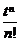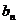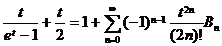To define the relationship between Bernoulli numbers and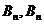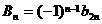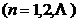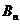For a table of numbers see Chapter V §3 , IX .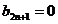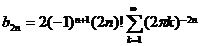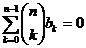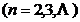2.        Bernoulli polynomial

function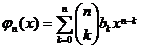(where is the Bernoulli number)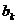is called a Bernoulli polynomial, which can also be expanded by the generating function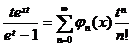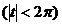to define .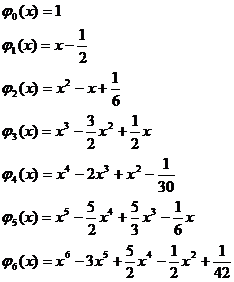·························································

The Bernoulli polynomial formula is as follows: